Journal of Integer Sequences, Vol. 13 (2010), Article 10.1.5

## Divisibility by 3 of Even Multiperfect Numbers of Abundancy 3 and 4

### Kevin A. Broughan and Qizhi Zhou University of Waikato Hamilton, New Zealand

Abstract:

We say a number is flat if it can be written as a non-trivial power of 2 times an odd squarefree number. The power is the exponent" and the number of odd primes the length". Let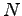be flat and 4-perfect with exponent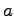and length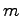. If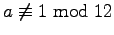, thenis even. Ifis even and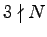thenis also even. If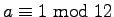then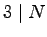andis even. Ifis flat and 3-perfect and, then if,is even. Ifthenis odd. Ifis flat and 3 or 4-perfect then it is divisible by at least one Mersenne prime, but not all odd prime divisors are Mersenne. We also give some conditions for the divisibility by 3 of an arbitrary even 4-perfect number.

Full version:  pdf,    dvi,    ps,    latex

(Concerned with sequences A005820 and A027687.)

Received June 30 2009; revised versions received October 12 2009; January 7 2010. Published in Journal of Integer Sequences, January 8 2010.